1. /
2. CBSE
3. /
4. Class 12
5. /
6. Physics
7. /
8. NCERT Solutions class 12...

# NCERT Solutions class 12 physics Wave Optics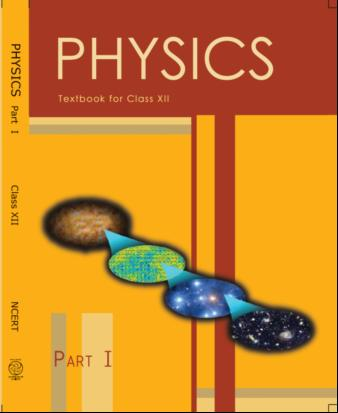## NCERT Class 12 Physics Chapter-wise Solutions

• 1 – Electric Charges and Fields
• 2 – Electrostatic Potential and Capacitance
• 3 – Current Electricity
• 4 – Moving Charges and Magnetism
• 5 – Magnetism and Matter
• 6 – Electromagnetic Induction
• 7 – Alternating Current
• 8 – Electromagnetic Waves
• 9 – Ray Optics and Optical Instruments
• 10 – Wave Optics
• 11 – Dual Nature of Radiation and Matter
• 12 – Atoms
• 13 – Nuclei
• 14 – Semiconductor Electronic: Material, Devices and Simple Circuits
• 15 – Communication Systems

## CHAPTER 10 WAVE OPTICS

• 10.1 Introduction
• 10.2 Huygens Principle
• 10.3 Refraction and reflection of plane waves using Huygens Principle
• 10.4 Coherent and Incoherent Addition of Waves
• 10.5 Interference of Light Waves and Young’s Experiment
• 10.6 Diffraction
• 10.7 Polarisation

## NCERT Solutions class-12 physics Wave Optics

1: Monochromatic light of wavelength 589 nm is incident from air on a water surface. What are the wavelength, frequency and speed of (a) reflected, and (b) refracted light? Refractive index of water is 1.33.

2: What is the shape of the wave front in each of the following cases:

(a) Light diverging from a point source.

(b) Light emerging out of a convex lens when a point source is placed at its focus.

(c) The portion of the wave front of light from a distant star intercepted by the Earth.

3: (a) The refractive index of glass is 1.5. What is the speed of light in glass? Speed of light in vacuum is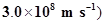(b) Is the speed of light in glass independent of the colour of light? If not, which of the two colours red and violet travels slower in a glass prism?

4:  In a Young’s double-slit experiment, the slits are separated by 0.28 mm and the screen is placed 1.4 m away. The distance between the central bright fringe and the fourth bright fringe is measured to be 1.2 cm. Determine the wavelength of light used in the experiment.

5: In Young’s double-slit experiment using monochromatic light of wavelength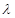, the intensity of light at a point on the screen where path difference is, is K units. What is the intensity of light at a point where path difference is/3?

6: A beam of light consisting of two wavelengths, 650 nm and 520 nm, is used to obtain interference fringes in a Young’s double-slit experiment.

(a) Find the distance of the third bright fringe on the screen from the central maximum for wavelength 650 nm.

(b) What is the least distance from the central maximum where the bright fringes due to both the wavelengths coincide?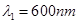7: In a double-slit experiment the angular width of a fringe is found to be 0.2° on a screen placed 1 m away. The wavelength of light used is 600 nm. What will be the angular width of the fringe if the entire experimental apparatus is immersed in water? Take refractive index of water to be 4/3.

8: What is the Brewster angle for air to glass transition? (Refractive index of glass = 1.5.)

9:  Light of wavelength 5000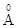falls on a plane reflecting surface. What are the wavelength and frequency of the reflected light? For what angle of incidence is the reflected ray normal to the incident ray?

10: Estimate the distance for which ray optics is good approximation for an aperture of 4 mm and wavelength 400 nm.

11: The 6563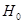line emitted by hydrogen in a star is found to be red shifted by 15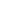¦. Estimate the speed with which the star is receding from the Earth.

12: Explain how Corpuscular theory predicts the speed of light in a medium, say, water, to be greater than the speed of light in vacuum. Is the prediction confirmed by experimental determination of the speed of light in water? If not, which alternative picture of light is consistent with experiment?

13: You have learnt in the text how Huygens’ principle leads to the laws of reflection and refraction. Use the same principle to deduce directly that a point object placed in front of a plane mirror produces a virtual image whose distance from the mirror is equal to the object distance from the mirror.

14: Let us list some of the factors, which could possibly influence the speed of wave propagation:

(i) Nature of the source.

(ii) Direction of propagation.

(iii) Motion of the source and/or observer.

(iv) Wave length.

(v) Intensity of the wave. On which of these factors, if any, does

(a) The speed of light in vacuum,

(b) The speed of light in a medium (say, glass or water), depend?

15:  For sound waves, the Doppler formula for frequency shift differs slightly between the two situations: (i) source at rest; observer moving, and (ii) source moving; observer at rest. The exact Doppler formulas for the case of light waves in vacuum are, however, strictly identical for these situations. Explain why this should be so. Would you expect the formulas to be strictly identical for the two situations in case of light travelling in a medium?

16: In double-slit experiment using light of wavelength 600 nm, the angular width of a fringe formed on a distant screen is 0.1º. What is the spacing between the two slits?

17: Answer the following questions: (a) In a single slit diffraction experiment, the width of the slit is made double the original width. How does this affect the size and intensity of the central diffraction band?

(b) In what way is diffraction from each slit related to the interference pattern in a double-slit experiment?

(c) When a tiny circular obstacle is placed in the path of light from a distant source, a bright spot is seen at the centre of the shadow of the obstacle. Explain why?

(d) Two students are separated by a 7 m partition wall in a room 10 m high. If both light and sound waves can bend around obstacles, how is it that the students are unable to see each other even though they can converse easily.

(e) Ray optics is based on the assumption that light travels in a straight line. Diffraction effects (observed when light propagates through small apertures/slits or around small obstacles) disprove this assumption. Yet the ray optics assumption is so commonly used in understanding location and several other properties of images in optical instruments. What is the justification?

18: Two towers on top of two hills are 40 km apart. The line joining them passes 50 m above a hill halfway between the towers. What is the longest wavelength of radio waves, which can be sent between the towers without appreciable diffraction effects?

19:A parallel beam of light of wavelength 500 nm falls on a narrow slit and the resulting diffraction pattern is observed on a screen 1 m away. It is observed that the first minimum is at a distance of 2.5 mm from the centre of the screen. Find the width of the slit.

(a) When a low flying aircraft passes overhead, we sometimes notice a slight shaking of the picture on our TV screen. Suggest a possible explanation.

(b) As you have learnt in the text, the principle of linear superposition of wave displacement is basic to understanding intensity distributions in diffraction and interference patterns. What is the justification of this principle?

21: In deriving the single slit diffraction pattern, it was stated that the intensity is zero at angles of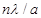. Justify this by suitably dividing the slit to bring out the cancellation.

## NCERT Solutions for Class 12 Physics

NCERT Solutions Class 12 Physics PDF (Download) Free from myCBSEguide app and myCBSEguide website. Ncert solution class 12 physics includes text book solutions from both part 1 and part 2. NCERT Solutions for CBSE Class 12 Physics have total 20 chapters. 12 Physics NCERT Solutions in PDF for free Download on our website. Ncert physics class 12 solutions PDF and physics ncert class 12 PDF solutions with latest modifications and as per the latest CBSE syllabus are only available in myCBSEguide

To download NCERT Solutions for class 12 Physics, Chemistry, Biology, History, Political Science, Economics, Geography, Computer Science, Home Science, Accountancy, Business Studies and Home Science; do check myCBSEguide app or website. myCBSEguide provides sample papers with solution, test papers for chapter-wise practice, NCERT solutions, NCERT Exemplar solutions, quick revision notes for ready reference, CBSE guess papers and CBSE important question papers. Sample Paper all are made available through the best app for CBSE students and myCBSEguide website.### Test Generator

Create question paper PDF and online tests with your own name & logo in minutes.### myCBSEguide

Question Bank, Mock Tests, Exam Papers, NCERT Solutions, Sample Papers, Notes